MATHEMATICS-2004

 Time: Two Hours (8.30 AM – 10.30 AM) Max. Marks: 60

NOTE:-

1. Attempt all questions.
2. Answer to each question under Sections D, E should begin on a fresh page and rough work must be enclosed with answer book.
3. While answering, refer to a question by its serial number as well as section heading (eg. Q2/Sec.E)
4. There is no negative marking.
5. Answer each of Sections A, B, C at one place.
6. Elegant solutions will be rewarded.
7. Use of calculators, slide rule, graph paper and logarithmic, trigonometric and statistical tables is not permitted.

Note:- All answers to questions in Section-A, Section-B, Section-C must be supported by mathematical arguments. In each of these sections order of the questions must be maintained.

SECTION-A

This section has Five Questions. Each question is provided with five alternative answers. Only one of them is the correct answer. Indicate the correct answer by A, B, C, D, E. (5x2=10 MARKS)

1. A lattice point is a point (x, y) in the plane such that x and y are integers. The number of rectangles with corners at lattice points, sides parallel to the axes, and center at the origin which have precisely 2004 lattice points on all of its sides put together is
 A) 0 B) 501 C) 499 D) 500 E) 2004
2. The number of positive integers which divide 2004 to leave a remainder of 24 is
3.  A) 36 B) 20 C) 22 D) 21 E) 34
4. ABCD is a parallelogram.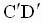is parallel to AB, with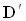and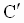in the interior of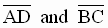respectively. Then
A) AC <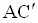is possible if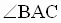is acute
B) AC <is possible ifis right
C) AC <wheneveris obtuse
D) AC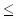wheneveris obtuse
E) none of these
5. a, b, c, d are real constants, x a real variable. Real numbers p and q exist such that p(ax+b) + q(cx+d) = ex+f
1. for every pair of reals (e, f)
2. for every pair of reals (e, f), if they exist for one pair of reals (e, f)
3. for every pair of reals (e, f), if they exist uniquely for one pair of reals (e, f)
4. for no pair of reals (e, f), if they do not exist for some pair of reals (e, f)
5. none of these
6. By a chord of the curve y = x3 we mean any line joining two distinct points on it. The number of chords which have slope –1 is
 A) infinite B) 0 C) 1 D) 2 E) None

SECTION-B

This section has Five Questions. In each question a blank is left. Fill in the blank. (5x2=10 MARKS)

1. The descending A.P. of 4 distinct positive integers with greatest possible last term and sum 2004 is __________
2. The radii of circles C1, C2, …., C2004 are respectively r1, r2, …., r2004. If r1 = 1 and ri = ri-1 + 1 for i = 2, 3,…..,2004, then r2004 = _________
3. f(n) =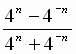for every integer n. p and q are integers such that p>q. The sign of f(p) – f(q) is _______
4. M1 is the initial point of a ray in a plane. Mi, for i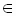{2, 3, ….,2004}, are points on the ray such that M1M2 = M2M3 = M3M4 = … = M2003M2004. M1 is (a,b) and M2004 is (c, d). If sx and sy are respectively the sum of all x-coordinates and the sum of all y-coordinates of Mi for i{1, 2, 3,….,2004}, then (sx, sy) = ______
5. The number of 2-element sets of nonunit positive integers such that their g.c.d. is 1 and l.c.m. is 2004 is ________

SECTION-C

 (4x2=8 MARKS)
1. Solve in positive integers x and y the equation x2 + y2 + 155(x+y)+2xy = 2004.
2.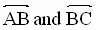are distinct lines. P is a point in the plane ABC and P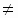B. Explain how to draw a line through P such that if the line intersects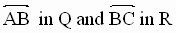, then BQ = BR.
3. Prove that there is no polynomial f(x) with integral coefficients such that f(1) = 2001 and f(3) = 2004.
4.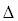ABC is right angled at B. A square is constructed on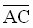on the side of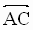opposite to that of B. P is the center of the square. Prove that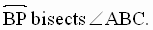SECTION-D

 (5x4=20 MARKS)
1. Evaluate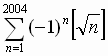, where [x] denotes the integral part of x.
2. ABCD is a line segment trisected by the points B, C; P is any point on the circle whose diameter is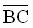. If the angles APB and CPD are respectively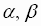, evaluate tan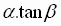.
3. Solve the system of equations in positive integers x, y, z:x2-y2+z2 = 2004, x + y – z = 48, xy – yz - zx = 125

4. a and b are positive reals and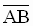a line segment in a plane. For how many distinct points C in the plane will it happen that for triangle ABC, the median and the altitude through C have lengths a and b respectively?
5. Prove by induction that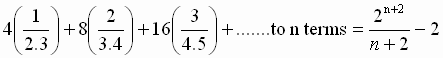SECTION-E

 (2x6=12 MARKS)
1. Find x if x+y+z+t = 1
x+3y+9z+27t = 81
x+4y+16z+64t = 256
x+167y+1672z+1673t = 1674
Hint: Avoid successive elimination of variables.

2. The perimeter of a triangle is 2004. One side of the triangle is 21 times the other. The shortest side is of integral length. Solve for lengths of the sides of the triangle in every possible case.